# Hyperspace

(diff) ← Older revision | Latest revision (diff) | Newer revision → (diff)
over a topological spaceA space whose points are the elements of some familyof subsets ofwith some topology.is usually a ring of sets, but this is not assumed in advance.
Example.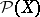is the hyperspace of all subsets of a space; a basis for the topology is formed by the sets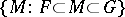provided thatis closed in,is open inand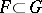.
The most frequently occurring hyperspace is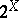. It consists of all closed subsets of a topological space; a sub-base of the exponential topology onis formed byand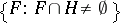, whereandare open inandruns through. Topologies in the following hyperspaces are defined in the same manner: on the set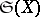of all compact subsets of a space; on the setof all finite subsets of a space; on the setof all subcontinua (connected compacta) of a continuum, etc. These spaces may be regarded as subspaces of the hyperspaceendowed with the exponential topology. Ifis a uniform space, then the sethas a natural uniform structure; the uniform space obtained in this way is denoted by. Ifis compact, then the hyperspaces,andare all homeomorphic and are compact. Ifis a compact metrizable space, so is. Ifis a continuum, so areand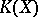.